# MA.5.DP.1.2Export Print
Interpret numerical data, with whole-number values, represented with tables or line plots by determining the mean, mode, median or range.

### Examples

Rain was collected and measured daily to the nearest inch for the past week. The recorded amounts are 1,0,3,1,0,0 and 1. The range is 3 inches, the modes are 0 and 1 inches and the mean value can be determined as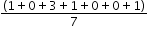which is equivalent to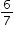of an inch. This mean would be the same if it rainedof an inch each day.

### Clarifications

Clarification 1: Instruction includes interpreting the mean in real-world problems as a leveling out, a balance point or an equal share.
General Information
Subject Area: Mathematics (B.E.S.T.)
Strand: Data Analysis and Probability
Status: State Board Approved

## Benchmark Instructional Guide

• Line Plots
• Mean
• Median
• Mode
• Range

### Vertical Alignment

Previous Benchmarks

Next Benchmarks

### Purpose and Instructional Strategies

The purpose of this benchmark is to interpret numerical data by using the mean, mode, median and range. This work builds on the previous understanding of mode, median, and range in Grade 4 (MA.4.DP.1.2). In Grade 6, a focus will be on comparing the advantages and disadvantages of the mean and median.
• When finding median and mode, it is important for students to organize their data, putting it in order from least to greatest.
• With the data organized, students can determine:
• range by subtracting the least value from the greatest value in the set.
• mode by finding the value that occurs most often.
• median by finding the value in middle of the set.
• mean by finding the average of the set of numbers.

### Common Misconceptions or Errors

• Students may confuse the mean and median of a data set. During instruction, teachers should provide students with examples where the median and mean of a data set are not close in value.

### Strategies to Support Tiered Instruction

• Instruction includes examples where the mean and the median are not close in value and uses a data set to explain the difference between mean and median.
• For example, the data set shown has a median of 4 and a mean of 7. The teacher uses the data to model how the mean is calculated and how the median is found.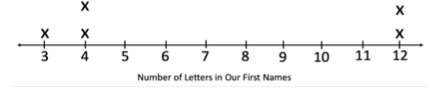• Instruction includes writing the data on index cards or sticky notes. Students can then easily arrange the data in order from least to greatest. This will assist in finding the median of the data set.
• For example, students use the data shown to explain the difference between mean (which is 7) and median (which is 4) and to model how the mean is calculated and how the median is found.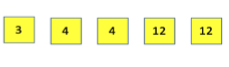Bobbie is a fifth grader who competes in the 100-meter hurdles. In her 8 track meets during the season, she recorded the following times to the nearest second.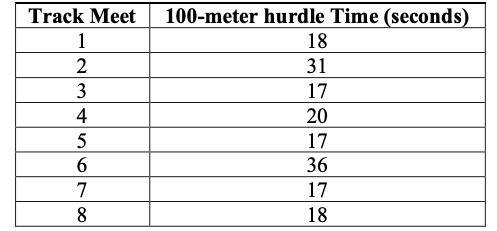• Part A. What is the mean time, in seconds, of Bobbie’s 100-meter hurdles?
• Part B. What is the median time, in seconds, of Bobbie’s 100-meter hurdles?
• Part C. What is the mode time, in seconds, of Bobbie’s 100-meter hurdles?
• Part D. If you were Bobbie, which of these results would you report to your friend?

### Instructional Items

Instructional Item 1

There was a pie-eating contest at the county fair. The line plot below shows the number of pies each of the 10 contestants ate. Use the line plot to determine the mean, mode, median and range of the data.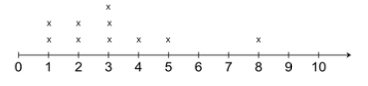*The strategies, tasks and items included in the B1G-M are examples and should not be considered comprehensive.

## Related Courses

This benchmark is part of these courses.
5012070: Grade Five Mathematics (Specifically in versions: 2014 - 2015, 2015 - 2022, 2022 and beyond (current))
7712060: Access Mathematics Grade 5 (Specifically in versions: 2014 - 2015, 2015 - 2018, 2018 - 2022, 2022 and beyond (current))
5012065: Grade 4 Accelerated Mathematics (Specifically in versions: 2019 - 2022, 2022 and beyond (current))
5012015: Foundational Skills in Mathematics 3-5 (Specifically in versions: 2019 - 2022, 2022 and beyond (current))

## Related Access Points

Alternate version of this benchmark for students with significant cognitive disabilities.
MA.5.DP.1.AP.2: Interpret numerical data, with whole-number values, represented with tables or line plots by determining the mean, mode or range. Line plot scales to include only whole numbers, halves and quarters.

## Related Resources

Vetted resources educators can use to teach the concepts and skills in this benchmark.

## Lesson Plans

Voting Rights Amendments: The Voting Rights Act of 1965:

In this lesson plan, students will create and analyze a line graph to explore how the Voting Rights Act of 1965 expanded civic participation.

Type: Lesson Plan

Voter Turnout and the 26th Amendment:

In this lesson plan, students will graph and interpret voter turnout data to explain how the 26th Amendment expanded the opportunity for civic participation.

Type: Lesson Plan

Voter Turnout and the 24th Amendment:

Students will use voter turnout data to create and analyze a line graph to explain how the 24th Amendment expanded civic participation in this integrated lesson plan.

Type: Lesson Plan

Practicing With Mean:

In this lesson plan, students will practice finding the mean of a set of data and be able to connect that to how mean is used in real-life. Students will be able to collect data, create line plots, interpret the data, and find the mean.

Type: Lesson Plan

Students will help the Supervisor of Elections determine which voter registration locations could be improved to help more citizens get registered to vote. Students will learn about the number of citizens who registered to vote in a general election year compared to the total population of those eligible to vote. They will discuss which voter registration locations will provide the most access to citizens and allocate funds to help address the issue in this modeling eliciting activity.

Type: Lesson Plan

Voter Turnout and the 19th Amendment:

Students will graph and analyze voting data to explain how the 19th Amendment expanded civic participation in this lesson plan.

Type: Lesson Plan

Voter Turnout and the 15th Amendment:

In this lesson plan, students will graph and analyze voting data to explain how the 15th Amendment expanded civic participation.

Type: Lesson Plan

How Does Force Affect Motion?:

Students will explore how force affects an object's motion. Students will discuss how the greater the mass of an object, the greater the force required to move an object.  Students will use data gathered through experimentation to justify their reasoning and understanding of forces and motion.

Type: Lesson Plan

Marbelous Pool Noodle Ramps:

In this lesson, students will build a ramp out of a pool noodle and use it to launch a marble across the room. Students will investigate by adjusting the height and slope of the ramp and record their findings on a data sheet. Students will practice collecting and analyzing data and will investigate the importance of performing repeated experimental trials. Students will practice converting metric units of distance as well as the addition and division of decimals to find the mean of a small data set.

Type: Lesson Plan

Paper Airplanes Away!:

In this lesson, students will design and fly their own paper airplane and analyze their flight data to determine the best designs for getting planes to travel the farthest distance. Students will organize class flight data into a line plot and calculate the mean, median, mode, and range for the data set.

Type: Lesson Plan

## Perspectives Video: Teaching Idea

Using Visual Models to Determine Mode, Median and Range:

Unlock an effective teaching strategy for teaching median, mode, and range in this Teacher Perspectives Video for educators.

Type: Perspectives Video: Teaching Idea

## Teaching Ideas

Ball Bounce Experiment:

Students investigate different balls' abilities to bounce and represent the data they collect graphically.

Type: Teaching Idea

Jump or Be Lunch! SeaWorld Classroom Activity:

Students will predict how high they can jump and then compare the height of their jumps to how high a rockhopper penguin can jump out of the water. They will practice mathematical skills for determining averages.

Type: Teaching Idea

## Tutorials

Redistributing Trail Mix:

This Khan Academy tutorial video presents a strategy for solving the following problem: given a dot plot with different measurements of trail mix in bags, find the amount of trail mix each bag would contain, if the total amount in all the bags was equally redistributed.

Type: Tutorial

Frequency tables and Dot Plots:

In this video, we organize data into frequency tables and dot plots (sometimes called line plots).

Type: Tutorial

## Student Resources

Vetted resources students can use to learn the concepts and skills in this benchmark.

## Tutorials

Redistributing Trail Mix:

This Khan Academy tutorial video presents a strategy for solving the following problem: given a dot plot with different measurements of trail mix in bags, find the amount of trail mix each bag would contain, if the total amount in all the bags was equally redistributed.

Type: Tutorial

Frequency tables and Dot Plots:

In this video, we organize data into frequency tables and dot plots (sometimes called line plots).

Type: Tutorial

## Parent Resources

Vetted resources caregivers can use to help students learn the concepts and skills in this benchmark.